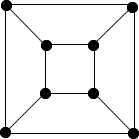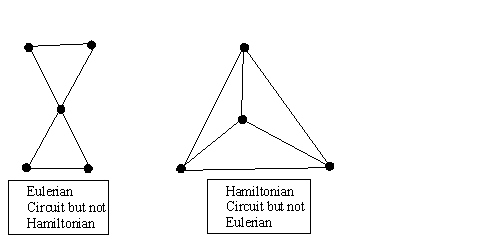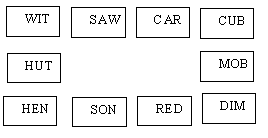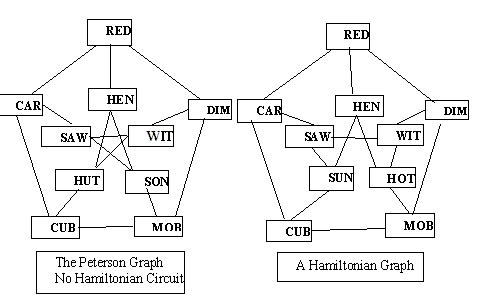# Some Circuits in Graph or Network Theory

##### Age 14 to 18

Published 2004 Revised 2012

A graph is a mathematical object made up of points (sometimes called nodes, see below) with lines joining some or all of the points. You can think of the world wide web as a graph. The points and lines are called vertices and edges just like the vertices and edges of polyhedra. Here is a graph representing a cube.Graph Theory is a whole mathematical subject in its own right, many books and papers are written on it and it is still an active research area with new discoveries still being made. Graphs are very useful in designing, representing and planning the use of networks (for example airline routes, electricity and water supply networks, delivery routes for goods, postal services etc.) Graphs are also used to solve problems in coding, telecommunications and parallel programming.

In some of these applications the actual distances and the geometrical shape of the graph is not important, simply which vertices in the system are linked, and these applications come into the branch of maths known as topology. In other applications distances between the vertices, the direction of flow and the capacity of the 'pipes' are significant. Rather confusingly there are two different languages used by mathematicians.  In this article we use the graph theory language.

 Graph Theory Graph Vertex Edge Degree Path Network Applications Network Node Arc Order Route

You can trace a path in the graph by taking a pencil, starting at one of the vertices and drawing some of the edges of the graph without lifting your pencil off the paper. A path is simply a sequence of vertices where each vertex is connected by a line to the next one in the sequence. A circuit is any path in the graph which begins and ends at the same vertex.

Two special types of circuits are Eulerian circuits, named after Leonard Euler (1707 to 1783), and Hamiltonian circuits named after William Rowan Hamilton (1805 to 1865). The whole subject of graph theory started with Euler and the famous Konisberg Bridge Problem.

An Eulerian circuit passes along each edge once and only once, and a Hamiltonian circuit visits each vertex once and only once. Both are useful in applications; the Hamiltonian circuits when it is required to visit each vertex (say every customer, every supply depot or every town) and the Eulerian circuits when it is required to travel along all the connecting edges (say all the streets in a town to collect the garbage). Note that for a Hamiltonian circuit it is not necessary to travel along each edge.

Here are two graphs, the first contains an Eulerian circuit but no Hamiltonian circuits and the second contains a Hamiltonian circuit but no Eulerian circuits. If you find it difficult to remember which is which just think E for edge and E for Euler. Can you draw for yourself other simple graphs which have one sort of circuit in them and not the other?Conditions for there to be Eulerian circuits are well know but in general it is a difficult problem to decide when a given graph has a Hamiltonian circuit. Finding conditions for the existence of Hamiltonian circuits is an unsolved problem.

The degree of a vertex is the number of edges joining onto that vertex, and vertices are said to be odd or even according to whether the degree is odd or even. Euler circuits exist only in networks where there are no odd vertices, that is where all the vertices have an even number of edges ending there.

Two edges are used each time the path visits and leaves a vertex because the circuit must use each edge only once. It follows that if the graph has an odd vertex then that vertex must be the start or end of the path and, as a circuit starts and ends at the same vertex, for a circuit to exist all the vertices must be even. When there are two odd vertices a walk can take place that traverses each edge exactly once but this will not be a circuit.

Can you think why it is impossible to draw any graph with an odd number of odd vertices (e.g. one odd vertex)? Well the reason is that each edge has two ends so the total number of endings is even, so the sum of the degrees of all the vertices in a graph must be even, so there cannot be an odd number of odd vertices.

Here is a simple puzzle, which we call the Prime Puzzle, for you to solve that uses and illustrates Hamiltonian circuits. Each of the following numbers is the product of exactly three prime factors and you have to arrange them in a sequence so that any two successive numbers in the sequence have exactly one common factor. The numbers are $222$, $255$, $385$, $874$, $2821$, $4199$, $11803$ and $20677$ and we have used only the first twelve prime numbers. First factorize the numbers, next start to draw the graph which will have $8$ vertices, one for each number. Take one number on a vertex and draw three edges from it and label them, one for each factor. Now attach the appropriate numbers at the ends of these edges. Repeat the procedure until the graph is complete. Any two vertices are joined by an edge if and only if they have a common factor. You should have eight vertices and twelve edges and this should suggest a neat way to draw the graph. You may wish to re-draw the graph so that the edges do not cross except at the eight vertices. The graph will be one where it is easy to find a Hamiltonian circuit and this circuit gives you the solution to the problem.Here is a similar but well known puzzle invented by Peterson where you have to arrange the ten cards in a loop so that each card has exactly one letter in common with each adjacent card. The words are HUT, WIT, SAW, CAR, CUB, MOB, DIM, RED, SON, HEN. The arrangement shown in the diagram looks very nearly correct but the words SON and RED do not match. If you try to solve the puzzle by re-arranging the cards you will not succeed because it is impossible. Now replace SON by SUN and HUT by HOT and the puzzle can be solved. The explanation is contained in the following two graphs.In the Peterson graph there are no Hamiltonian circuits so, unlike the Primes Puzzle above there is no way to put the cards into the required circuit. Changing two of the cards to SON and HUT makes it possible to find a Hamiltonian circuit and solve the problem.

On the NRICH website you will find a lot of problems on graphs and networks which you might like to try. Also why not do some research on the web and find out about Euler and Hamilton, both giants in the mathematical world.